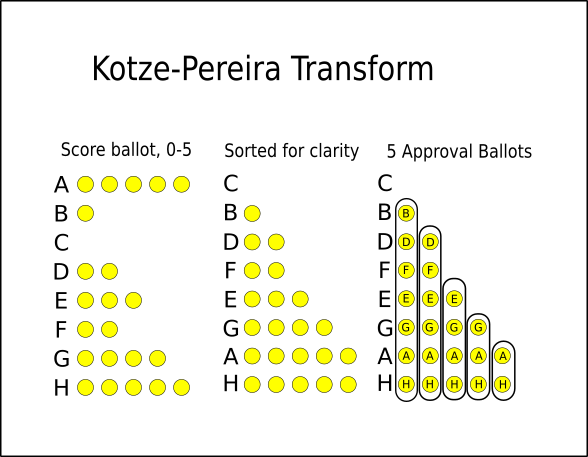# Technically Exists

## Sequential proportional score voting

### Introduction

Sequential proportional score voting (SPSV) is a rated party-agnostic proportional method based on sequential proportional approval voting (SPAV) and the Kotze-Pereira transformation (KP transform). For this reason, it is often referred to as SPAV + KP.

### Description

Voters cast ballots by rating all candidates on a scale such as 0 to 5, where a blank rating is interpreted as the minimum value. Each rated ballot is converted into five virtual approval ballots using the KP transform, as shown below.Once this is done, SPAV is run on the virtual approval ballots to determine which candidates are elected. Each virtual ballot starts off with a weight of 1. For each seat, the candidate with the most weighted approvals is elected, and every virtual ballot is given a weight of 1/(1 + m), where m is the number of candidates approved on the ballot who have already been elected.

### Analysis

With the above reweighting formula, SPSV is equivalent to D’Hondt when voters vote perfectly along party lines. However, if the formula 1/(1 + 2m) is used instead, then under such circumstances it becomes equivalent to Webster/Sainte-Laguë.

SPSV is similar to Reweighted Range Voting (RRV), but it has the additional benefit of passing scale invariance.

When voters min-max vote, SPSV reduces to SPAV. Equivalently, SPAV and SPSV with a scale of 0 to 1 are mathematically identical and give the same results.